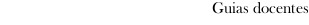Guia docente 2023_24Facultade de Ciencias Económicas e Empresariais
 Grado en EconomíaSubjectsEconometrics IContents
 Topic Sub-topic TOPIC 1: Empirical questions and the problem of causal inference. Econometric models - Types of empirical questions and examples. - The problem of causal inference. - Methods for estimating causal effects. TOPIC 2: Linear regression model (I) - Selection on observables. - Linear regression model: specification. - Ordinary Least Squares estimation. - Interpretation of estimates: when do we identify a causal effect? TOPIC 3: Linear regression model (II) - Goodness of fit. - The random component of the Ordinary Least Squares estimator. - Assumptions of the regression model. - Precision of the estimates. - Properties of the Ordinary Least Squares estimator. TOPIC 4: Linear regression model (III) - Obtaining additional information from the parameter estimates: changes of scale of the variables; elasticities; Beta coefficients. - Non-linearities between the dependent variable and the explanatory variables of the model. TOPIC 5: Inference in the linear regression model - Assumption of normality. - Hypothesis testing on a single parameter. - Confidence intervals. - Contrasts of multiple restrictions on the parameters. TOPIC 6: Dummy variables - A single independent dummy variable. - Dummy variables for multiple categories - Interactions involving dummy variables. TOPIC 7: Specification and data problems in regression analysis - Consequences of misspecification of the functional form. - Omission of relevant variables. - Inclusion of irrelevant variables. - Multicollinearity TOPIC 8: Heteroscedasticity - Detection - Consequences - Solutions TOPIC 9: Correlation of error terms across observations - Detection - Consequences - Solution TOPIC 10: Endogeneity - Causes of endogeneity: omitted variable bias, measurement error in explanatory variables, bidirectional causality. - Instrumental variables estimation.
 Universidade de Vigo            | Reitoría | Campus Universitario | C.P. 36.310 Vigo (Pontevedra) | España | Tlf: +34 986 812 000